# PSAT Math : Geometry

## Example Questions

### Example Question #1 : How To Find The Length Of The Side Of A Square

When the side of a certain square is increased by 2 inches, the area of the resulting square is 64 sq. inches greater than the original square.  What is the length of the side of the original square, in inches?

17

14

15

18

16

15

Explanation:

Let x represent the length of the original square in inches.  Thus the area of the original square is x2.  Two inches are added to x, which is represented by x+2.  The area of the resulting square is (x+2)2.  We are given that the new square is 64 sq. inches greater than the original.  Therefore  we can write the algebraic expression:

x2 + 64 = (x+2)2

FOIL the right side of the equation.

x2 + 64 = x2 + 4x + 4

Subtract xfrom both sides and then continue with the alegbra.

64 = 4x + 4

64 = 4(x + 1)

16 = x + 1

15 = x

Therefore, the length of the original square is 15 inches.

If you plug in the answer choices, you would need to add 2 inches to the value of the answer choice and then take the difference of two squares.  The choice with 15 would be correct because 172 -152 = 64.

### Example Question #1 : How To Find The Length Of The Side Of A Square

If the area of a square is 25 inches squared, what is the perimeter?

25

15

10

20

Not enough information

20

Explanation:

The area of a square is equal to length times width or length squared (since length and width are equal on a square). Therefore, the length of one side is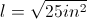or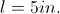The perimeter of a square is the sum of the length of all 4 sides or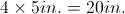### Example Question #1 : Rectangles

What is the length of the diagonal of a rectangle that is 3 feet long and 4 feet wide?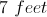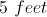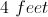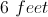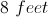Explanation:

The diagonal of the rectangle is equivalent to finding the length of the hypotenuse of a right triangle with sides 3 and 4. Using the Pythagorean Theorem: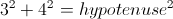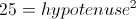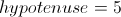Therefore the diagonal of the rectangle is 5 feet.

### Example Question #1 : How To Find The Length Of The Diagonal Of A Rectangle

The length and width of a rectangle are in the ratio of 3:4. If the rectangle has an area of 108 square centimeters, what is the length of the diagonal?

9 centimeters

24 centimeters

18 centimeters

12 centimeters

15 centimeters

15 centimeters

Explanation:

The length and width of the rectangle are in a ratio of 3:4, so the sides can be written as 3x and 4x.

We also know the area, so we write an equation and solve for x:

(3x)(4x) = 12x= 108.

x2 = 9

x = 3

Now we can recalculate the length and the width:

length = 3x = 3(3) = 9 centimeters

width = 4x = 4(3) = 12 centimeters

Using the Pythagorean Theorem we can find the diagonal, c:

length2 + width2 = c2

92 + 12= c2

81 + 144 = c2

225 = c2

= 15 centimeters

### Example Question #1 : How To Find The Length Of The Side Of A Rectangle

The two rectangles shown below are similar. What is the length of EF?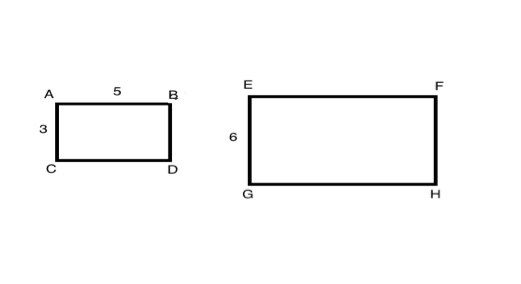10

8

6

5

10

Explanation:

When two polygons are similar, the lengths of their corresponding sides are proportional to each other.  In this diagram, AC and EG are corresponding sides and AB and EF are corresponding sides.

To solve this question, you can therefore write a proportion:

AC/EG = AB/EF ≥ 3/6 = 5/EF

From this proportion, we know that side EF is equal to 10.

### Example Question #42 : Quadrilaterals

A rectangle is x inches long and 3x inches wide.  If the area of the rectangle is 108, what is the value of x?

3

6

12

4

8

6

Explanation:

Solve for x

Area of a rectangle A = lw = x(3x) = 3x2 = 108

x2 = 36

x = 6

### Example Question #43 : Quadrilaterals

If the area of rectangle is 52 meters squared and the perimeter of the same rectangle is 34 meters. What is the length of the larger side of the rectangle if the sides are integers?

13 meters

14 meters

15 meters

12 meters

16 meters

13 meters

Explanation:

Area of a rectangle is = lw

Perimeter = 2(l+w)

We are given 34 = 2(l+w) or 17 = (l+w)

possible combinations of l + w

are 1+16, 2+15, 3+14, 4+13... ect

We are also given the area of the rectangle is 52 meters squared.

Do any of the above combinations when multiplied together= 52 meters squared? yes 4x13 = 52

Therefore the longest side of the rectangle is 13 meters

### Example Question #6 : Rectangles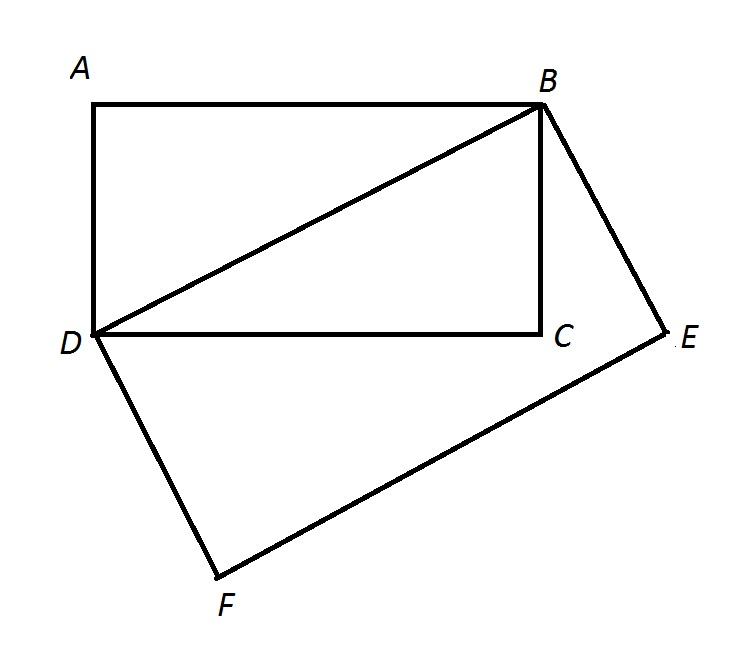Note: Figure NOT drawn to scale.

In the above figure,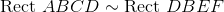.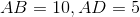.

Give the perimeter of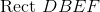.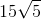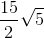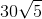Explanation:

We can use the Pythagorean Theorem to find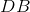: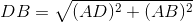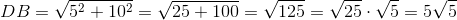The similarity ratio ofto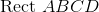is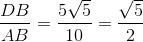so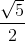multiplied by the length of a side ofis the length of the corresponding side of. We can subsequently multiply the perimeter of the former byto get that of the latter: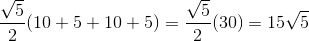### Example Question #7 : RectanglesNote: Figure NOT drawn to scale.

In the above figure,.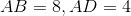.

Give the area of.Insufficient information is given to determine the area.Explanation:

Corresponding sidelengths of similar polygons are in proportion, so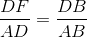, so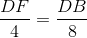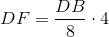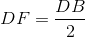We can use the Pythagorean Theorem to find: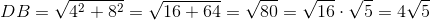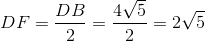The area ofis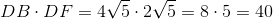### Example Question #1 : How To Find If Rectangles Are SimilarNote: Figure NOT drawn to scale.

In the above figure,.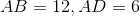.

Give the area of Polygon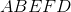.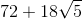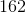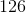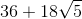Explanation:

Polygoncan be seen as a composite of right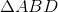and, so we calculate the individual areas and add them.

The area ofis half the product of legs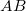and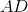: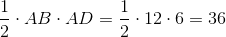Now we find the area of. We can do this by first findingusing the Pythagorean Theorem: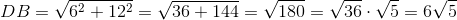The similarity oftoimpliesso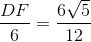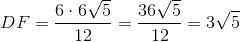The area ofis the product ofand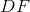: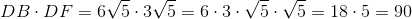Now add: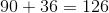, the correct response.

### All PSAT Math Resources